# Creating a YARA Rule to Detect Obfuscated Strings

Published: 2023-09-04
Last Updated: 2023-09-04 08:55:33 UTC
by Didier Stevens (Version: 1)
2 comment(s)

I wrote a blog post "Quickpost: Analysis of PDF/ActiveMime Polyglot Maldocs" on how to analyse PDF/ActiveMime polyglot malicious document files and also developed a YARA rule to detect them.

These polyglot files are PDF files into which an Office document (for example, a Single File Web Page Word document) has been embedded (in various ways).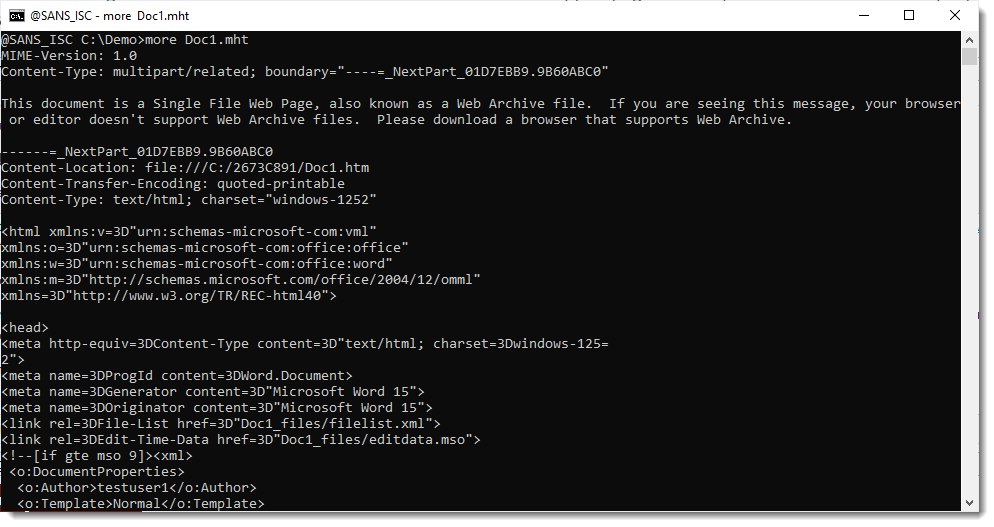When a Word document with VBA code is saved as a Single File Web Page, the ole file with the VBA code is stored inside an ActiveMime file. That file starts with binary sequence ActiveMime.

A Single File Web Page is a MIME file, the ActiveMime file is stored as a BASE64 encoded part.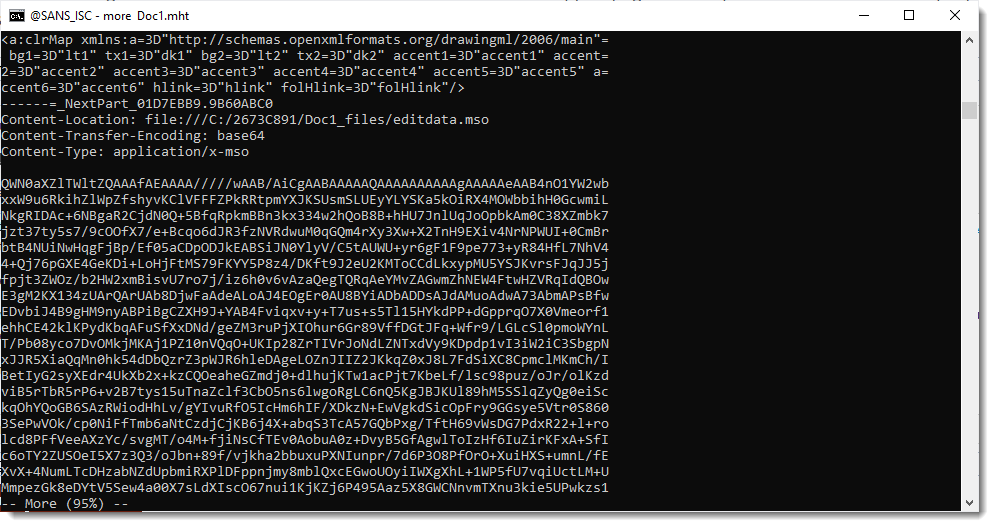To detect PDF/ActiveMime maldocs, I developed a YARA rule that looks for a PDF header at the start of the file and contains the string "ActiveMime" in BASE64.

A simple rule to implement this looks like this:

``````rule pdfactivemime1 {
strings:
\$pdf = "%PDF-"
\$activemime = "ActiveMime" base64
condition:
\$pdf at 0 and \$activemime
}
``````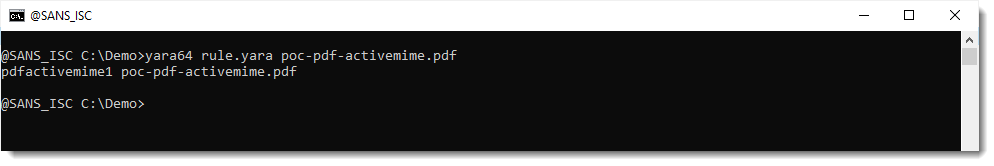A simple rule like that is able to detect the simple PoC file I made, but is not able to detect the maldocs found in the wild. That's because of obfuscation.

The BASE64 encoded part in the MIME file can be obfuscated by adding extra whitespace characters between the individual characters that compose the BASE64 string.

Here is a simple example. ActiveMim in BASE64 is QWN0aXZlTWlt. I added an extra line break between Q and WN0aXZlTWlt: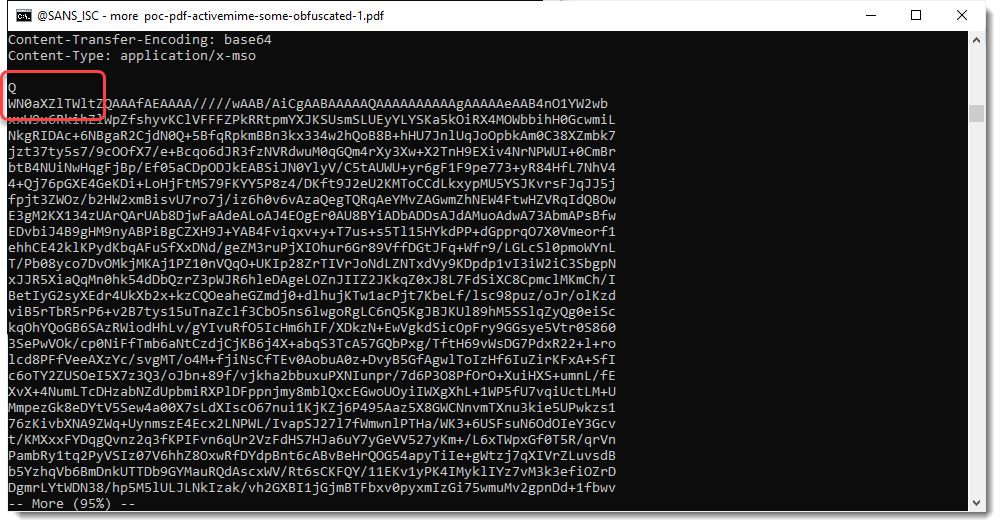This simple change breaks the simple YARA rule, but does not hinder the Word parser: it is still able to read the document.

In the maldocs found in-the-wild, much more whitespace characters were added. So we need a YARA rule to take this into account, and the solution is to use a regular expression that looks for the characters QWN0aXZlTWlt (potentialy) interspersed with whitespace characters (I selected 4 whitespace characters: space, tab, carriage-return and newline).

Like this rule:

``````rule pdfactivemime2 {
strings:
\$pdf = "%PDF-"
\$activemime = /Q[ \t\r\n]*W[ \t\r\n]*N[ \t\r\n]*0[ \t\r\n]*a[ \t\r\n]*X[ \t\r\n]*Z[ \t\r\n]*l[ \t\r\n]*T[ \t\r\n]*W[ \t\r\n]*l[ \t\r\n]*t/
condition:
\$pdf at 0 and \$activemime
}
``````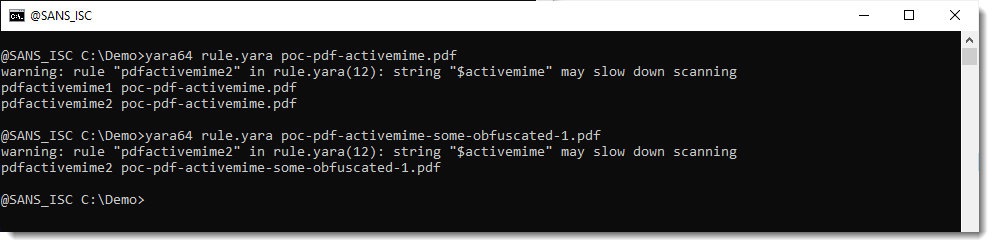The rule works, however, YARA issues a warning, that the rule may slow down scanning.

So you can use this rule for ad-hoc detection, but not for intensive scanning.

The problem is the following: one of the first steps of a YARA scan, is to search for so called "atoms". Atoms are short, fixed substrings of the strings you provide in your rules.

The fixed substrings in string \$activemime in rule pdfactivemime2 are all individual characters, e.g., they are one byte long. And that is too short to execute a performant Aho-Corasick search (the search algorithm used by the YARA engine).

The solution I implemented is the following: I made a set of regular expressions that all start with 3 fixed characters, by generating all the possible combinations (product):

• Q followed by 2 space characters
• Q followed by a space character and a tab character
• Q followed by a space character and a carriage-return character
• ...
• Q followed by W and a space character (e.g., no whitespace between Q and W)
• ...

Just a single regex needs to match for the rule to trigger:

``````rule rule_pdf_activemime {
meta:
author = "Didier Stevens"
date = "2023/08/29"
version = "0.0.1"
description = "look for files that start with %PDF- and contain BASE64 encoded string ActiveMim (QWN0aXZlTWlt), possibly obfuscated with extra whitespace characters"
usage = "if you don't have to care about YARA performance warnings, you can uncomment string \$base64_ActiveMim0 and remove all other \$base64_ActiveMim## strings"
strings:
\$pdf = "%PDF-"
\$base64_ActiveMim1 = /Q  [ \t\r\n]*W[ \t\r\n]*N[ \t\r\n]*0[ \t\r\n]*a[ \t\r\n]*X[ \t\r\n]*Z[ \t\r\n]*l[ \t\r\n]*T[ \t\r\n]*W[ \t\r\n]*l[ \t\r\n]*t/
\$base64_ActiveMim2 = /Q \t[ \t\r\n]*W[ \t\r\n]*N[ \t\r\n]*0[ \t\r\n]*a[ \t\r\n]*X[ \t\r\n]*Z[ \t\r\n]*l[ \t\r\n]*T[ \t\r\n]*W[ \t\r\n]*l[ \t\r\n]*t/
\$base64_ActiveMim3 = /Q \r[ \t\r\n]*W[ \t\r\n]*N[ \t\r\n]*0[ \t\r\n]*a[ \t\r\n]*X[ \t\r\n]*Z[ \t\r\n]*l[ \t\r\n]*T[ \t\r\n]*W[ \t\r\n]*l[ \t\r\n]*t/
\$base64_ActiveMim4 = /Q \n[ \t\r\n]*W[ \t\r\n]*N[ \t\r\n]*0[ \t\r\n]*a[ \t\r\n]*X[ \t\r\n]*Z[ \t\r\n]*l[ \t\r\n]*T[ \t\r\n]*W[ \t\r\n]*l[ \t\r\n]*t/
\$base64_ActiveMim5 = /Q\t [ \t\r\n]*W[ \t\r\n]*N[ \t\r\n]*0[ \t\r\n]*a[ \t\r\n]*X[ \t\r\n]*Z[ \t\r\n]*l[ \t\r\n]*T[ \t\r\n]*W[ \t\r\n]*l[ \t\r\n]*t/
\$base64_ActiveMim6 = /Q\t\t[ \t\r\n]*W[ \t\r\n]*N[ \t\r\n]*0[ \t\r\n]*a[ \t\r\n]*X[ \t\r\n]*Z[ \t\r\n]*l[ \t\r\n]*T[ \t\r\n]*W[ \t\r\n]*l[ \t\r\n]*t/
\$base64_ActiveMim7 = /Q\t\r[ \t\r\n]*W[ \t\r\n]*N[ \t\r\n]*0[ \t\r\n]*a[ \t\r\n]*X[ \t\r\n]*Z[ \t\r\n]*l[ \t\r\n]*T[ \t\r\n]*W[ \t\r\n]*l[ \t\r\n]*t/
\$base64_ActiveMim8 = /Q\t\n[ \t\r\n]*W[ \t\r\n]*N[ \t\r\n]*0[ \t\r\n]*a[ \t\r\n]*X[ \t\r\n]*Z[ \t\r\n]*l[ \t\r\n]*T[ \t\r\n]*W[ \t\r\n]*l[ \t\r\n]*t/
\$base64_ActiveMim9 = /Q\r [ \t\r\n]*W[ \t\r\n]*N[ \t\r\n]*0[ \t\r\n]*a[ \t\r\n]*X[ \t\r\n]*Z[ \t\r\n]*l[ \t\r\n]*T[ \t\r\n]*W[ \t\r\n]*l[ \t\r\n]*t/
\$base64_ActiveMim10 = /Q\r\t[ \t\r\n]*W[ \t\r\n]*N[ \t\r\n]*0[ \t\r\n]*a[ \t\r\n]*X[ \t\r\n]*Z[ \t\r\n]*l[ \t\r\n]*T[ \t\r\n]*W[ \t\r\n]*l[ \t\r\n]*t/
\$base64_ActiveMim11 = /Q\r\r[ \t\r\n]*W[ \t\r\n]*N[ \t\r\n]*0[ \t\r\n]*a[ \t\r\n]*X[ \t\r\n]*Z[ \t\r\n]*l[ \t\r\n]*T[ \t\r\n]*W[ \t\r\n]*l[ \t\r\n]*t/
\$base64_ActiveMim12 = /Q\r\n[ \t\r\n]*W[ \t\r\n]*N[ \t\r\n]*0[ \t\r\n]*a[ \t\r\n]*X[ \t\r\n]*Z[ \t\r\n]*l[ \t\r\n]*T[ \t\r\n]*W[ \t\r\n]*l[ \t\r\n]*t/
\$base64_ActiveMim13 = /Q\n [ \t\r\n]*W[ \t\r\n]*N[ \t\r\n]*0[ \t\r\n]*a[ \t\r\n]*X[ \t\r\n]*Z[ \t\r\n]*l[ \t\r\n]*T[ \t\r\n]*W[ \t\r\n]*l[ \t\r\n]*t/
\$base64_ActiveMim14 = /Q\n\t[ \t\r\n]*W[ \t\r\n]*N[ \t\r\n]*0[ \t\r\n]*a[ \t\r\n]*X[ \t\r\n]*Z[ \t\r\n]*l[ \t\r\n]*T[ \t\r\n]*W[ \t\r\n]*l[ \t\r\n]*t/
\$base64_ActiveMim15 = /Q\n\r[ \t\r\n]*W[ \t\r\n]*N[ \t\r\n]*0[ \t\r\n]*a[ \t\r\n]*X[ \t\r\n]*Z[ \t\r\n]*l[ \t\r\n]*T[ \t\r\n]*W[ \t\r\n]*l[ \t\r\n]*t/
\$base64_ActiveMim16 = /Q\n\n[ \t\r\n]*W[ \t\r\n]*N[ \t\r\n]*0[ \t\r\n]*a[ \t\r\n]*X[ \t\r\n]*Z[ \t\r\n]*l[ \t\r\n]*T[ \t\r\n]*W[ \t\r\n]*l[ \t\r\n]*t/
\$base64_ActiveMim17 = /QW [ \t\r\n]*N[ \t\r\n]*0[ \t\r\n]*a[ \t\r\n]*X[ \t\r\n]*Z[ \t\r\n]*l[ \t\r\n]*T[ \t\r\n]*W[ \t\r\n]*l[ \t\r\n]*t/
\$base64_ActiveMim18 = /QW\t[ \t\r\n]*N[ \t\r\n]*0[ \t\r\n]*a[ \t\r\n]*X[ \t\r\n]*Z[ \t\r\n]*l[ \t\r\n]*T[ \t\r\n]*W[ \t\r\n]*l[ \t\r\n]*t/
\$base64_ActiveMim19 = /QW\r[ \t\r\n]*N[ \t\r\n]*0[ \t\r\n]*a[ \t\r\n]*X[ \t\r\n]*Z[ \t\r\n]*l[ \t\r\n]*T[ \t\r\n]*W[ \t\r\n]*l[ \t\r\n]*t/
\$base64_ActiveMim20 = /QW\n[ \t\r\n]*N[ \t\r\n]*0[ \t\r\n]*a[ \t\r\n]*X[ \t\r\n]*Z[ \t\r\n]*l[ \t\r\n]*T[ \t\r\n]*W[ \t\r\n]*l[ \t\r\n]*t/
\$base64_ActiveMim21 = /QWN[ \t\r\n]*0[ \t\r\n]*a[ \t\r\n]*X[ \t\r\n]*Z[ \t\r\n]*l[ \t\r\n]*T[ \t\r\n]*W[ \t\r\n]*l[ \t\r\n]*t/
condition:
\$pdf at 0 and any of (\$base64_ActiveMim*)
}``````

By hardcoding the first 3 character combinations in each regex, the YARA engine can select atoms that are larger than one byte (3 bytes maximum in this case).

You can find this rule in my GitHub repository here and the PoCs in my GitHub repository here.

Remark that the maldocs in-the-wild have more obfuscation methods (like the MIME-Version header) and that it is possible to significantly reduce the MIME file in size.

PDF files need to start with header %PDF- at the first position in the file according to the PDF language specification, but PDF parsers are lenient and the %PDF- header can be located later in the file. The YARA rule I made does not account for this.

Didier Stevens
Senior handler
Microsoft MVP
blog.DidierStevens.com

Keywords:
2 comment(s)